### IMO Shortlist 1974 problem 4

Kvaliteta:
Avg: 0,0
Težina:
Avg: 0,0
The sum of the squares of five real numbers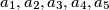$a_1, a_2, a_3, a_4, a_5$ equals$1$. Prove that the least of the numbers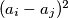$(a_i - a_j)^2$, where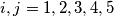$i, j = 1, 2, 3, 4,5$ and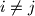$i \neq j$, does not exceed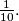$\frac{1}{10}.$
Izvor: Međunarodna matematička olimpijada, shortlist 1974